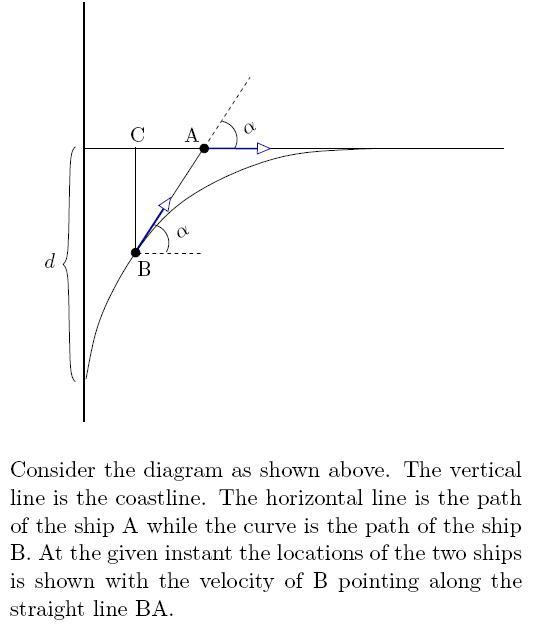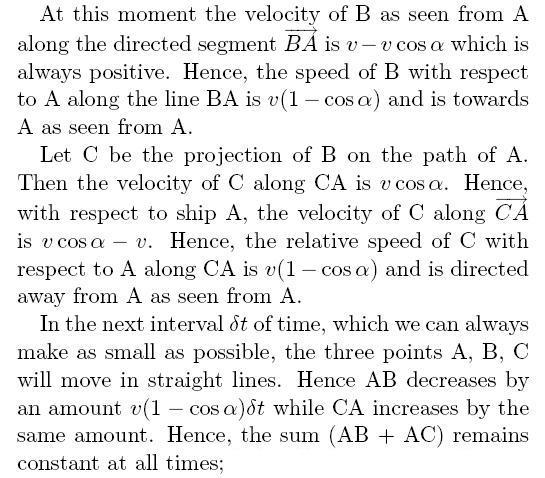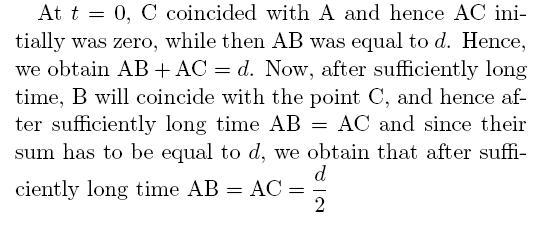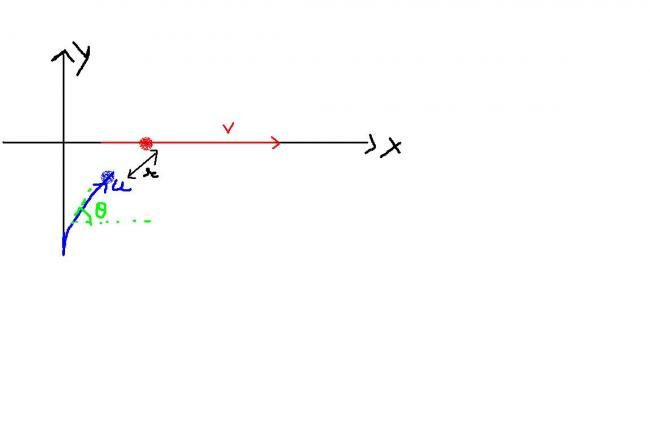# Kaymant Sir's question relative motion

Q1. Two ships A and B originally at a distance d = 3 km from each other depart at the same time from a straight coastline. Ship A moves along a straight line perpendicular to the shore while ship B constantly heads for ship A, having at each moment the same speed as the later. After a suffciently large interval of time, ship B will obviously follow ship A at a certain distance. Find this distance.

A more generalised one...

Q2. Two particles A & B start from positions ( 0, 0 ) & ( 0, -d ) and move with constant speeds v & u respectively . A moves along x - axis and B moves such that its velocity is always aimed at A . Let r be the distance between them and be the angle made by the velocity of B with X - axis , at some time t .

Prove that , r/d = [(1-cos\theta )^u^/^v]/[(sin\theta )^u^/^v^+^1]

•satan92 ·

d/2=1.5 not 1/5 as mentioned sry

•Jijo Sagai ·

How come vcosA - v = v(1 - cosA)??? In Vca?

•eureka123 ·

•eureka123 ·

sir pehle to iska matlab bataoo......"""the concept of invariance.""'

•kaymant ·

eureka123, kaun sa argument samajh nahi aaya?

•satan92 ·

great solution!

•eureka123 ·

sorry sir par kuch samajh nahin aaya.....

•kaymant ·

This was my solution to the first part: the concept of invariance.•satan92 ·

the second one is even easier!

let the angle at any instant be Î¸ to the horizontal

then we have

dr/dt=vcosÎ¸-u

and

dÎ¸/dt=-vsinÎ¸/r

so dt=dr/vcosÎ¸-u

(u-vcosÎ¸)dÎ¸/vsinÎ¸=dr/r

integrating we get .

log(cosecÎ¸-cotÎ¸)u/v - logsinÎ¸=log(r/d)

we get

r/d=(cosecÎ¸-cotÎ¸)u/v/(sinÎ¸)

thus

r/d=(1-cosÎ¸)u/v/(sinÎ¸)(u/v)+1

•Abhishek Priyam ·

Ship B sees that it is heading to A or ship A sees so or observer from ground see that...

I think ship B sees that...

•satan92 ·

the first one is not lengthy at all ..

we have if the line joining the two particles makes an angle Î¸ with the horizontal ..

and if the distance between them is r

dr/dt=(ucosÎ¸-u)

intial r=d final let D

w get
D-d=0âˆ«tucosÎ¸dt -0âˆ«tudt

further we have

final distance D also= âˆ«udt-âˆ«ucosÎ¸dt

so we get D-d=-D

D=d/2 =1/5

•eureka123 ·

ok sir........will try to do further.......

vaise what are its chances of coming in JEE??
becoz it looks a bit lengthy.......

it tried to form a 3-4 eqn but nothing gained out of them

•kaymant ·

yes the situation is like what you have shown..

•eureka123 ·

anyways 2 things are clear........
dx/dt = u cosÎ¸
and dy/dt = usinÎ¸

what next????

•eureka123 ·

At time t is the situation like this??????If yes then i will try to do it

•Asish Mahapatra ·

ans is correct for 1st question .. provide solutions ...

•king_khan ·

ans : 3/2 ie 1.5 km ????

•